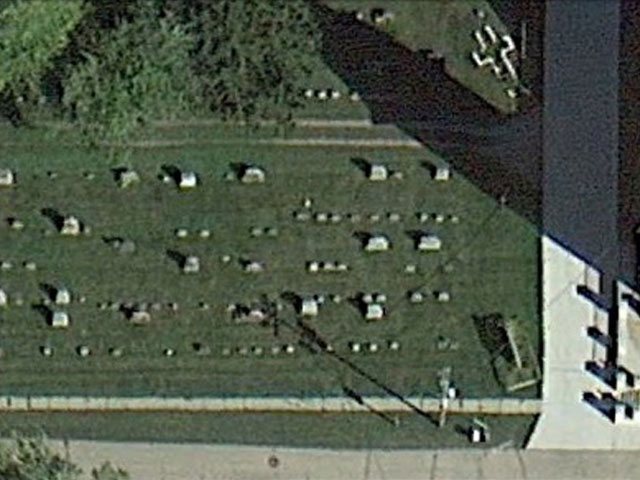# GPR Survey Time can be Estimated

## The GPR survey time is only a guesstimate

### but it is better than nothing.

According to Sensors & Software, manufacturers of ground penetrating radar, there are several variables to the speed of a survey. The biggest factor is collection speed, which is most affected by terrain and obstacles. Obviously, a survey over a groomed golf course will go quicker than through heavy bush.

### What is the GPR survey time for a single GPR line?

Over a smooth surface and without stopping, an average person can walk about 2 miles per hour (find out more about walking), without missing traces. That is, about 10,000 linear feet per hour can be covered at a reasonable walking pace with GPR. Taking into account the GPR system needs to be moved to the start of new line and repositioned, let’s assume that it takes twice as long, thus, roughly 5,000 linear feet per hour. Let’s call this V, for velocity or the apparent walking speed.

### How much area does each line effectively cover?

This depends on the line spacing. Lets call this W.  A tighter line spacing, smaller W, means more lines are needed to cover the same area, which obviously means it will take more time to complete a survey. Line spacing is an important aspect of collecting data. One rule of thumb suggest the line spacing should be a maximum of an antenna’s width apart (as if you were cutting you lawn.) A wider line spacing may result in incomplete data and poor post processing results. If W is 2 feet then the GPR systems covers approximately V*W. For example, 5,000 linear feet per hour by 2 feet, or 10,000 square feet per hour. In contrast, if the line spacing is 1 foot wide at 5,000 feet per hour, then 5,000 square feet per hour may be covered and if the line spacing is 0.25 feet (3 inches) then about 1,250 square feet are covered per hour.

### How large is my survey area?

Now that you know about how much area you can survey per hour you need to consider the size of the site. A 100 by 100 foot (10,000 square feet) site is four times larger than a 50 by 50 foot site (2,500 square feet). To determine the area of the grid multiply the length of the X axis, call this X, times the length of the Y axis, call this Y. If a grid with perpendicular lines is being used then multiply the area time 2, because you have to go over the site twice and the GPR survey time will be twice as long. For example, the X axis is 200 feet long (X=200 ft) and the Y axis is 50 feet in length (Y=50). The site is then X*Y or 200 x 50 square feet or 10,000 square feet. If a grid with X and Y lines is acquired than the apparent size of the site is 2*X*Y or 20,000 square feet.

### So what is the GPR survey time for a grid?

Obviously, you now know now the GPR survey time depends on how large the GPR grid is and how far apart the lines are spaced. All that there is left in the calculation is to divide the apparent size of the site by the apparent rate the GPR can acquire data.

Estimated GPR Survey Time = (X * Y) / (V * W)

for one pass over the survey area or multiply this time 2 if acquiring perpendicular lines.

From above a 50 by 200 ft site with lines space 2 feet apart walking at an apparent speed of 5000 feet per hour would take approximately

Estimated GPR Survey Time = 1 grid * (50 ft x 200 ft / (5000 feet per hour * 2 feet) or 1 hour

One more example from above, say the site is 50 by 50 ft site with perpendicular lines spaced 0.25 feet apart walking at an apparent speed of 5000 feet per hour would take approximately

Estimated GPR Survey Time = 2 grids * (50 ft x 50 ft / (5000 feet per hour * 0.25 feet) or 4 hours

### Why did the GPR survey time take so much longer?

First of all, most things look better on paper than they do once you have done the job. Remember, you must take in to account the actual speed you can walk a site, obstacles, the time it takes to set up the grid, the time used to talk with interested persons, breaks, and mistakes (as if that never happens).